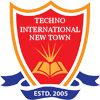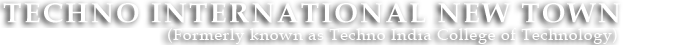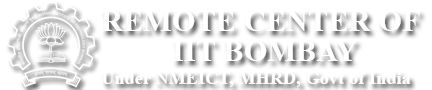#+91 9674112076/2079
••••# Circuit Theory Lab

PC-EE-391 (3rd Semester)

Major Equipments:

• DSO, CRO, Milli-voltmeter and ammeter, Digital meter, Analog meter, multi-meter, LC meter
• Function Generator, True AC milli-voltmeter, True AC milli-ammeter,
• RLC trainer Kit, RC and RL trainer kit
• Passive low pass and High pass filter kit,
• Active low pass, High pass, band pass & band reject filter kit,
• Trainer kit for measurement of Impedance (Z) and Admittance (Y) parameter of two-port network
• MATLAB (Software)

Experiment List:

• Transient response of R-L and R-C network: simulation with software & hardware
• Transient response of R-L-C series and parallel circuit: simulation with software & hardware
• Determination of Impedance (Z) and Admittance (Y) parameter of two-port network: simulation & hardware.
• Frequency response of LP and HP filters: simulation & hardware.
• Frequency response of BP and BR filters: simulation & hardware.
• Generation of Periodic, Exponential, Sinusoidal, Damped Sinusoidal, Step, Impulse, Ramp signal using MATLAB in both discrete and analog form.
• Determination of Laplace transform and Inverse Laplace transform using MATLAB.
• Amplitude and Phase spectrum analysis of different signals using MATLAB.
• Verification of Network theorems using software & hardware.

Beyond the Curriculum:

• Generation of matrices and its operation in MATLAB.